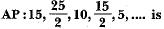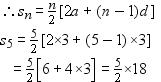Courses

# RS Aggarwal Test: Arithmetic Progressions

## 10 Questions MCQ Test Mathematics (Maths) Class 10 | RS Aggarwal Test: Arithmetic Progressions

Description
This mock test of RS Aggarwal Test: Arithmetic Progressions for Class 10 helps you for every Class 10 entrance exam. This contains 10 Multiple Choice Questions for Class 10 RS Aggarwal Test: Arithmetic Progressions (mcq) to study with solutions a complete question bank. The solved questions answers in this RS Aggarwal Test: Arithmetic Progressions quiz give you a good mix of easy questions and tough questions. Class 10 students definitely take this RS Aggarwal Test: Arithmetic Progressions exercise for a better result in the exam. You can find other RS Aggarwal Test: Arithmetic Progressions extra questions, long questions & short questions for Class 10 on EduRev as well by searching above.
QUESTION: 1

### The 11th and 13th terms of an AP are 35 and 41 respectively, its common difference is

Solution:

11th term is 35 so a+10d = 35 => equation 1

13th term is 41 so a+12d = 41 => equation 2

solve both equations by elimination method

we get common difference as 3

QUESTION: 2

### An AP consists of 31 terms. If its 16th term is m, then sum of all the terms of this AP is

Solution:

S31 = 31/2(2a + 30d)
a16 = a + 15d = m
⇒ S31 = (31/2) x 2(a + 15d) ⇒ S31 = 31 m

QUESTION: 3

### Which term of the AP: 18, 23, 28, 33,... is 98?

Solution:

98=a+(n-1)d

98=18+(n-1)5

80=(n-1)5

16=n-1

17=n

this explains that 98 is 17th term of the AP

QUESTION: 4

The 16th term of theSolution:

A= 15
d = a2 - a1
d = 25 / 2 - 15
d = ( 30 - 25 / 2 )
d = - 5/ 2
16th term of an AP = a + 15 d
15 +( 15 × ( -5 / 2 ))
15 + (-75 / 2 )
= - 45 / 2

QUESTION: 5

The list of numbers -10, -6, -2, 2,... is

Solution:

a1=-10
a2=-6
so the common difference
d=(-6-(-10)) = 4

QUESTION: 6

The sum of first 16 terms of the AP : 10, 6, 2,... is

Solution:

The formula for the sum of first n terms of a AP is n/2(2a+(n-1)d)
here n=16 a=10 d=6-10=2-6=-4
so sum of first 16 terms=16/2(2·10+(16-1)·-4)
=8(20+15·-4)
=8(20-60)
=8(-40)
=-320

QUESTION: 7

The sum of first five multiples of 3 is

Solution:

First five multiple of 3 are:

3, 6, 9, 12, 15

Here first term, a = 3

common difference, d = 6 – 3 = 3

Number of term, n = 5⇒ s5 = 9 × 5 = 45

QUESTION: 8

Two APs have same common difference. The first term of one of these is -1 and that of the other is - 8. Then the difference between their 4th term is

Solution:

Correct Answer :- c

Explanation : ad1 = -1

ad2 = -8

4th term of ad1 is

a4 = a + 3d

a4 = -1 + 3d.....(1)

Similarly ad2 is

a4 = -8 + 3d.......(2)

Subtracting (2) from (1), we get

-1 + 3d -(-8 + 3d)

=> 7

QUESTION: 9

The 21st term of the AP whose first two terms are -3 and 4, is

Solution:

a1=-3,
a2=4,
d=4-(-3)=7,
a21=?,
a21=a+(n-1)d
=-3+(21-1)7
= -3+(20)(7)
= -3+140
=137

QUESTION: 10

If 18, a, b, -3 are in AP, then a + b is equal to

Solution:

we know

AM  is ( a + b)/2

(a+b) = [ {18+(-3) }/2] X 2 =15